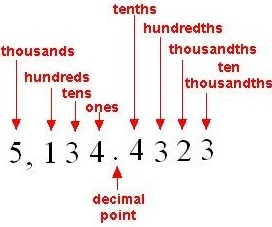# Decimal Place Value: Hundreds to Ten Thousandths

#### Complete Python Prime Pack

9 Courses     2 eBooks

#### Artificial Intelligence & Machine Learning Prime Pack

6 Courses     1 eBooks

#### Java Prime Pack

9 Courses     2 eBooks

The value of a digit based on its position or place in a number is known as its place value.

Consider the number 5,134.4323. The place value names of different digits in the number are shown in the figure below.Here 4 is in ones place; 3 is in tens place; 1 is in hundreds place; 5 is in thousands place; 4 is in tenths place; 3 is in hundredths place; 2 is in thousandths place; and 3 is in ten thousandths place.

### Rules for Decimal place values

The first digit to the left of decimal point is in the ones place.

The second digit to the left of decimal point is in the tens place.

The third digit to the left of decimal point is in the hundreds place and so on.

The first digit to the right of decimal point is in the tenths place.

The second digit to the right of decimal point is in the hundredths place.

The third digit to the right of decimal point is in the thousandths place.

The fourth digit to the right of decimal point is in the ten thousandths place and so on.

Give the digits in ones place and tenths place in the number 64.89

### Solution

Step 1:

In the given number 64.89, the digit in ones place is 4 as it is the first digit to the left of decimal point.

Step 2:

The digit in tenths place is 8 as it is the first digit to the right of decimal point.

Give the digits in tens place and hundredths place in the number 567.03

### Solution

Step 1:

In the given number 567.03, the digit in tens place is 6 as it is the second digit to the left of decimal point.

Step 2:

The digit in hundredths place is 3 as it is the second digit to the right of decimal point.

Give the digits in hundreds place and ten thousandths place in the number 932.1087

### Solution

Step 1:

In the given number 932.108, the digit in hundreds place is 9 as it is the third digit to the left of decimal point.

Step 2:

The digit in ten thousandths place is 7 as it is the fourth digit to the right of decimal point.